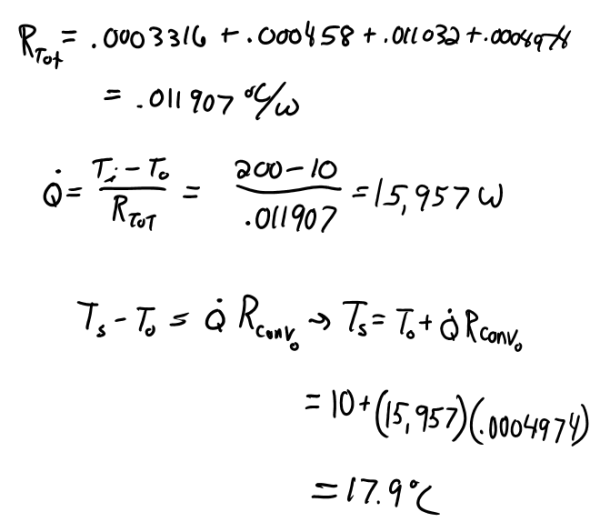heat and mass transfer problems engineering equations heat and mass transfer school homework engineering heat and mass transfer formulas heat and mass transfer solutions to heat and mass transfer problems full solution engineering problem solution heat and mass transfer math problems engineering equations heat and mass transfer school homework engineering solutions to heat and mass transfer formulas heat problems mass problem solutions to transfer problems full solution heat and mass transfer
heat and mass transfer problems engineering equations heat and mass transfer school homework engineering heat and mass transfer formulas heat and mass transfer solutions to heat and mass transfer problems full solution engineering problem solution heat and mass transfer math problems engineering equations heat and mass transfer school homework engineering solutions to heat and mass transfer formulas heat problems mass problem solutions to transfer problems full solution heat and mass transfer
Highalphabet Home Page heat and mass transfer problem solutions Heat and Mass Transfer Page
Superheated steam at an average temperature 200 C is transported through a steel pipe (k=50 W/mK, D_0=8.0 cm,D_i=6.0 cm,and L=20.0 m). The pipe is insulated with a 4-cm thick layer of gypsum plaster (k=0.5 W/mK). The insulated pipe is placed horizontally inside a warehouse where the average air temperature is 10 C. The steam and the air heat transfer coefficients are estimated to be 800 and 200 W/m^2 K, respectively. Calculate (a) the daily rate of heat transfer from the superheated steam, and (b) the temperature on the outside surface of the gypsum plaster insulation.Superheated steam at an average temperature 200 C is transported through a steel pipe (k=50 W/mK, D_0=8.0 cm,D_i=6.0 cm,and L=20.0 m). The pipe is insulated with a 4-cm thick layer of gypsum plaster (k=0.5 W/mK). The insulated pipe is placed horizontally inside a warehouse where the average air temperature is 10 C. The steam and the air heat transfer coefficients are estimated to be 800 and 200 W/m^2 K, respectively. Calculate (a) the daily rate of heat transfer from the superheated steam, and (b) the temperature on the outside surface of the gypsum plaster insulation.